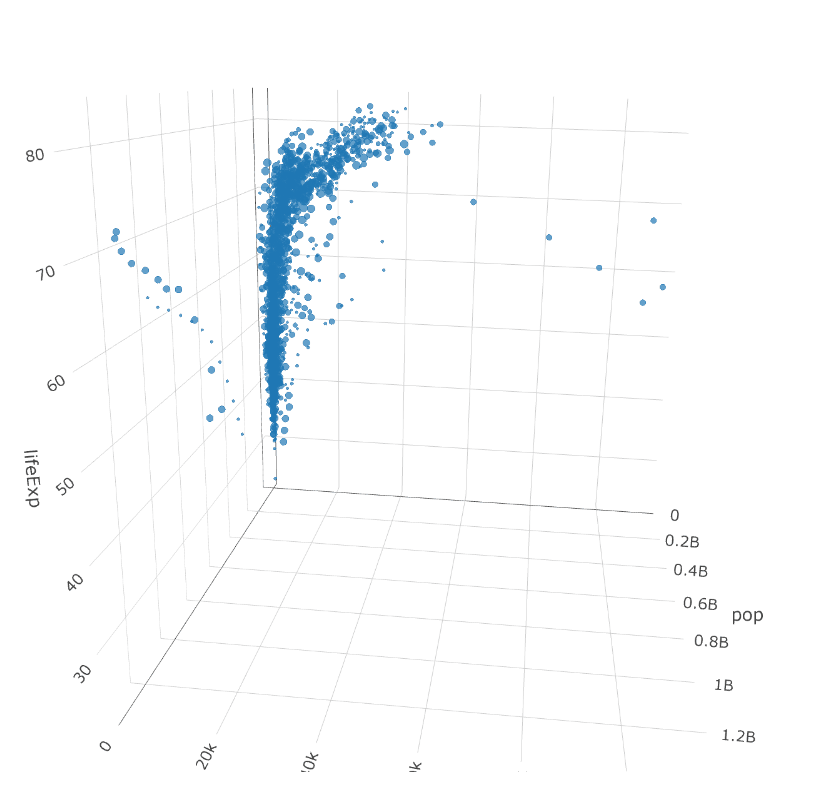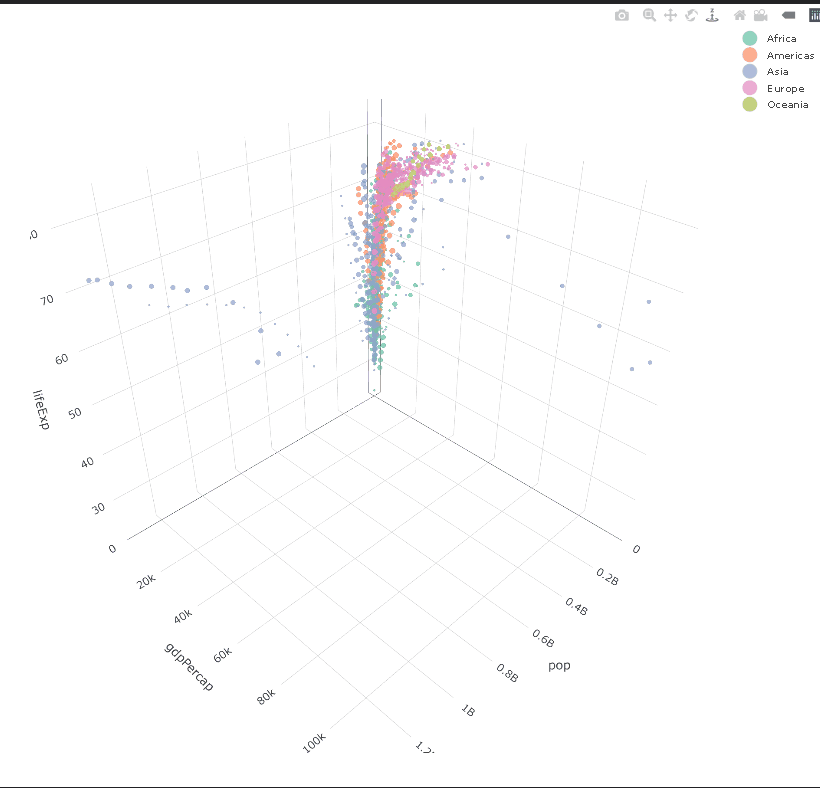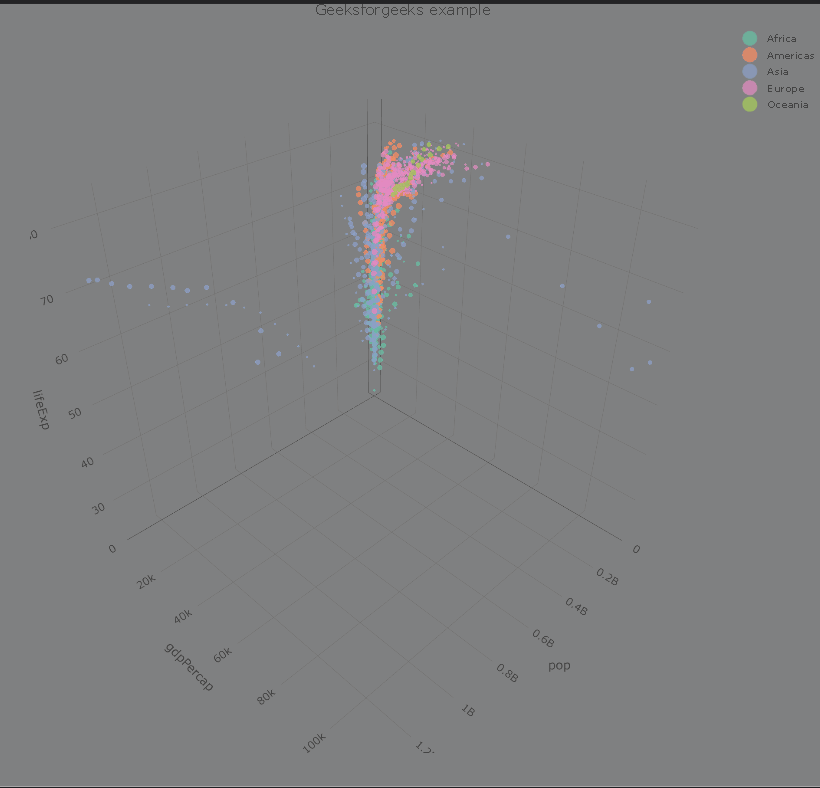# 3D bubble plot in R

In this article, we will discuss how to create a 3D bubble plot in the R Language.

A bubble chart is primarily used to depict and show relationships between numeric variables. It can show a relationship better than a scatter plot because it has an extra dimension size that helps us in analyzing one more aspect of data thus making it multi-dimensional analysis.

`Install  - install.packages("Plotly")`

The plot_ly() function creates a 3D scatter plot but by adding size dimension to it.

Syntax:

plot_ly(df, x, y, z, color, size)

where,

• df: determines the data frame to be used.
• x,y, and z: determine the x-axis, y-axis, and z-axis variables respectively.
• color: determines the categorical variable according to which bubbles are to be colored.
1. size: determines the categorical variable according to which bubbles are to be sized.

Example 1:

Here, is a basic 3D bubble plot. The CSV file used in the example can be downloaded here.

## R

 `# load library Plotly and tidyverse ` `library``(plotly) ` `library``(tidyverse) ` ` `  `# read csv file into dataframe ` `df <- ``read.csv``(``"df.csv"``) ` ` `  `# plot 3d bubble plot ` `plot_ly``(df, x = ~pop, y = ~gdpPercap, z = ~lifeExp, size = ~size )`

Output:Example 2:

Here, is a basic 3D bubble plot colored by a categorical variable using the color parameter of plot_ly() function. The CSV file used in the example can be downloaded here.

## R

 `# load library Plotly and tidyverse ` `library``(plotly) ` `library``(tidyverse) ` ` `  `# read csv file into dataframe ` `df <- ``read.csv``(``"df.csv"``) ` ` `  `# plot 3d bubble plot ` `# color plot by continent column using color parameter ` `plot_ly``(df, x = ~pop, y = ~gdpPercap, z = ~lifeExp, size = ~size, color=~continent )`

Output:## Customization of 3D plot

We can customize this 3D plot using various parameters of the layout function of the plot. We can use paper_bgcolor, plot_bgcolor, and title parameter to change the color of the surrounding of the plot, background color of plot, and the title of the plot respectively.

Syntax:

plot%>%layout( title, paper_bgcolor, plot_bgcolor)

where,

• title: determines the title of the plot.
• paper_bgcolor: determines the color of the surrounding of the plot.
• plot_bgcolor: determines the color of the background of the plot.

Example 1:

Here is a completely customized 3D bubble plot. The CSV file used in the example can be downloaded here.

## R

 `# load library Plotly and tidyverse ` `library``(plotly) ` `library``(tidyverse) ` ` `  `# read csv file into dataframe ` `df <- ``read.csv``(``"df.csv"``) ` ` `  `# plot 3d bubble plot ` `# color plot by continent column using  ` `# color parameter ` `plot<-``plot_ly``(df, x = ~pop, y = ~gdpPercap, z = ~lifeExp,  ` `              ``size = ~size, color=~continent) ` ` `  `# layout customization is used to customize plot ` `plot%>% ``layout``( title = ``'Geeksforgeeks example'``, ` `               ``paper_bgcolor = ``'gray'``)`

Output:Whether you're preparing for your first job interview or aiming to upskill in this ever-evolving tech landscape, GeeksforGeeks Courses are your key to success. We provide top-quality content at affordable prices, all geared towards accelerating your growth in a time-bound manner. Join the millions we've already empowered, and we're here to do the same for you. Don't miss out - check it out now!

Previous
Next